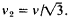# Second Sound

Also found in: Medical, Wikipedia.

## Second sound

A type of wave propagated in the superfluid phase of liquid helium (helium II) and in certain other substances under special conditions. The name is misleading since second sound is not in any sense a sound wave, but a temperature or entropy wave. In ordinary or first sound, pressure and density variations propagate with very small accompanying variations in temperature; in second sound, temperature variations propagate with no appreciable variation in density or pressure. See Liquid helium, Superfluidity

The two-fluid model of helium II provides further insight into the nature of second sound. In this model the liquid can be described as consisting of superfluid and normal components of densities ρs and ρn, respectively, such that the total density ρ = ρs + ρn. The superfluid component is frictionless and devoid of entropy; the normal component has a normal viscosity and contains the entropy and thermal energy of the system. In a temperature or second-sound wave, the normal and superfluid flows are oppositely directed so that ρs V s + ρn V n = 0, where V s and V n are the superfluid and normal flow velocities. Thus a variation in relative densities of the two components, and hence a temperature fluctuation, propagates with no change in total density or pressure. In a first-sound wave, the two components move in phase, that is, V nV s.

Theoretical predictions that second sound should exist in certain solid dielectric crystals under suitable conditions have been confirmed experimentally for solid helium single crystals at temperatures between 0.4 and 1.0 K (-459.0 and -457.9°F). See Dielectric materials

Another quite different class of materials can exhibit second sound. In smectic A liquid crystals, when the wave vector is oblique with respect to the layers of these ordered structures, a modulation of the interlayer spacing can propagate at nearly constant density.

## Second Sound

weakly attenuated temperature waves that are propagated in superfluid liquid helium (He II) along with ordinary sound waves. Near a temperature of absolute zero the velocity v2 of second sound and the velocity v of ordinary sound (compression waves) are associated by the ratioAt the point of phase transition from He II to He I (the A-point), v2 vanishes. Second sound is radiated from a heater with a variable temperature and is detected by a sensitive thermometer.

## second sound

[′sek·ənd ′sau̇nd]
(acoustics)
A transverse sound wave which propagates in smectic liquid crystals, and whose behavior resembles mathematically that of second sound in superfluid helium.
(cryogenics)
A type of wave propagated in the superfluid phase of liquid helium (helium II), in which temperature and entropy variations propagate with no appreciable variation in density or pressure.
References in periodicals archive ?
One of which, called thermoelasticity with second sound, suggests the replacement of Fourier's law by so called Cattaneo's law.
Results concerning existence and asymptotic behavior of smooth as well as weak solutions in thermoelasticity with second sound have been established by many mathematicians.
In the same year as this cataract of glass, Regli staged a second sound composition using the sirens and foghorns of seven motorboats and a steamship on the Lake of Zurich (Reality Hacking Nr.
Had the traditional press silenced the candidates' voices by compressing their views into eight or 12 second sound bites?

Site: Follow: Share:
Open / Close